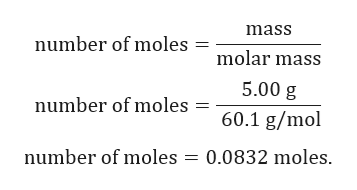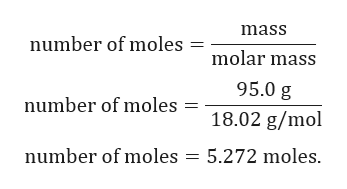# What is the mole fraction of acetic acid in vinegar? Vinegar is anaqueous solution that contains 5.00 % by mass acetic acid. Acetic acid isCH3COOH and has a molar mass of 60.1 g/mol.

Question
68 views
What is the mole fraction of acetic acid in vinegar? Vinegar is an
aqueous solution that contains 5.00 % by mass acetic acid. Acetic acid is
CH3COOH and has a molar mass of 60.1 g/mol.
check_circle

Step 1

Given:

5.00% by mass acetic acid in vinegar.

Molar mass of acetic acid = 60.1 g/mol.

Assume that we have 100 g of solution.

Solution will contain 5.00 g of acetic acid and 95.0 g of water.

Step 2

Calculation for number of moles of acetic acid:help_outlineImage Transcriptionclosemass number of moles molar mass 5.00 g 60.1 g/mol number of moles = number of moles = 0.0832 moles. fullscreen
Step 3

Calculation for number o...help_outlineImage Transcriptionclosemass number of moles molar mass 95.0 g number of moles 18.02 g/mol number of moles 5.272 moles fullscreen

### Want to see the full answer?

See Solution

#### Want to see this answer and more?

Solutions are written by subject experts who are available 24/7. Questions are typically answered within 1 hour.*

See Solution
*Response times may vary by subject and question.
Tagged in

### Solutions